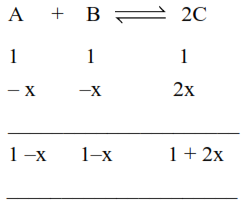# For the reaction

Question:

For the reaction

$\mathrm{A}+\mathrm{B} \rightleftharpoons 2 \mathrm{C}$

the value of equilibrium constant is 100 at $298 \mathrm{~K}$.

If the initial concentration of all the three species is

$1 \mathrm{M}$ each, then the equilibrium concentration of $\mathrm{C}$ is $x \times 10^{-1}$ M. The value of $x$ is______

(Nearest integer)

Solution:$\mathrm{K}=\frac{[\mathrm{C}]_{\mathrm{eq}}^{2}}{[\mathrm{~A}]_{\mathrm{eq}}[\mathrm{B}]_{\mathrm{eq}}}=\frac{(1+2 \mathrm{x})^{2}}{(1-\mathrm{x})(1-\mathrm{x})}$

$100=\left(\frac{1+2 x}{1-x}\right)^{2}$

$\left(\frac{1+2 x}{1-x}\right)=10$

$x=\frac{3}{4}$

$[\mathrm{C}] \mathrm{e}_{\mathrm{q} .}=1+2 \mathrm{x}$

$=1+2\left(\frac{3}{4}\right)$

$=2.5 \mathrm{M}$

$25 \times 10^{-1} \mathrm{M}$